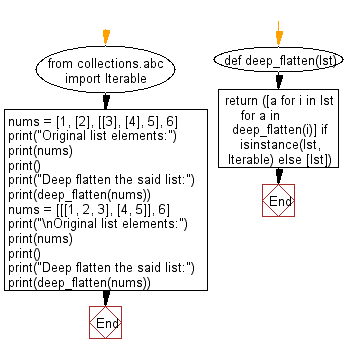﻿ Python: Perform a deep flattens a list - w3resource# Python: Perform a deep flattens a list

## Python List: Exercise - 255 with Solution

Write a Python program to perform a deep flattens a list.

• Use recursion.
• Use isinstance() with collections.abc.Iterable to check if an element is iterable.
• If it is iterable, apply deep_flatten() recursively, otherwise return [lst].

Sample Solution:

Python Code:

``````from collections.abc import Iterable
def deep_flatten(lst):
return ([a for i in lst for a in
deep_flatten(i)] if isinstance(lst, Iterable) else [lst])
nums = [1, , [, , 5], 6]
print("Original list elements:")
print(nums)
print()
print("Deep flatten the said list:")
print(deep_flatten(nums))
nums = [[[1, 2, 3], [4, 5]], 6]
print("\nOriginal list elements:")
print(nums)
print()
print("Deep flatten the said list:")
print(deep_flatten(nums))
```
```

Sample Output:

```Original list elements:
[1, , [, , 5], 6]

Deep flatten the said list:
[1, 2, 3, 4, 5, 6]

Original list elements:
[[[1, 2, 3], [4, 5]], 6]

Deep flatten the said list:
[1, 2, 3, 4, 5, 6]
```

Flowchart:## Visualize Python code execution:

The following tool visualize what the computer is doing step-by-step as it executes the said program:

Python Code Editor:

Have another way to solve this solution? Contribute your code (and comments) through Disqus.

What is the difficulty level of this exercise?

Test your Python skills with w3resource's quiz

﻿

## Python: Tips of the Day

```print(2_000_000)
```2000000